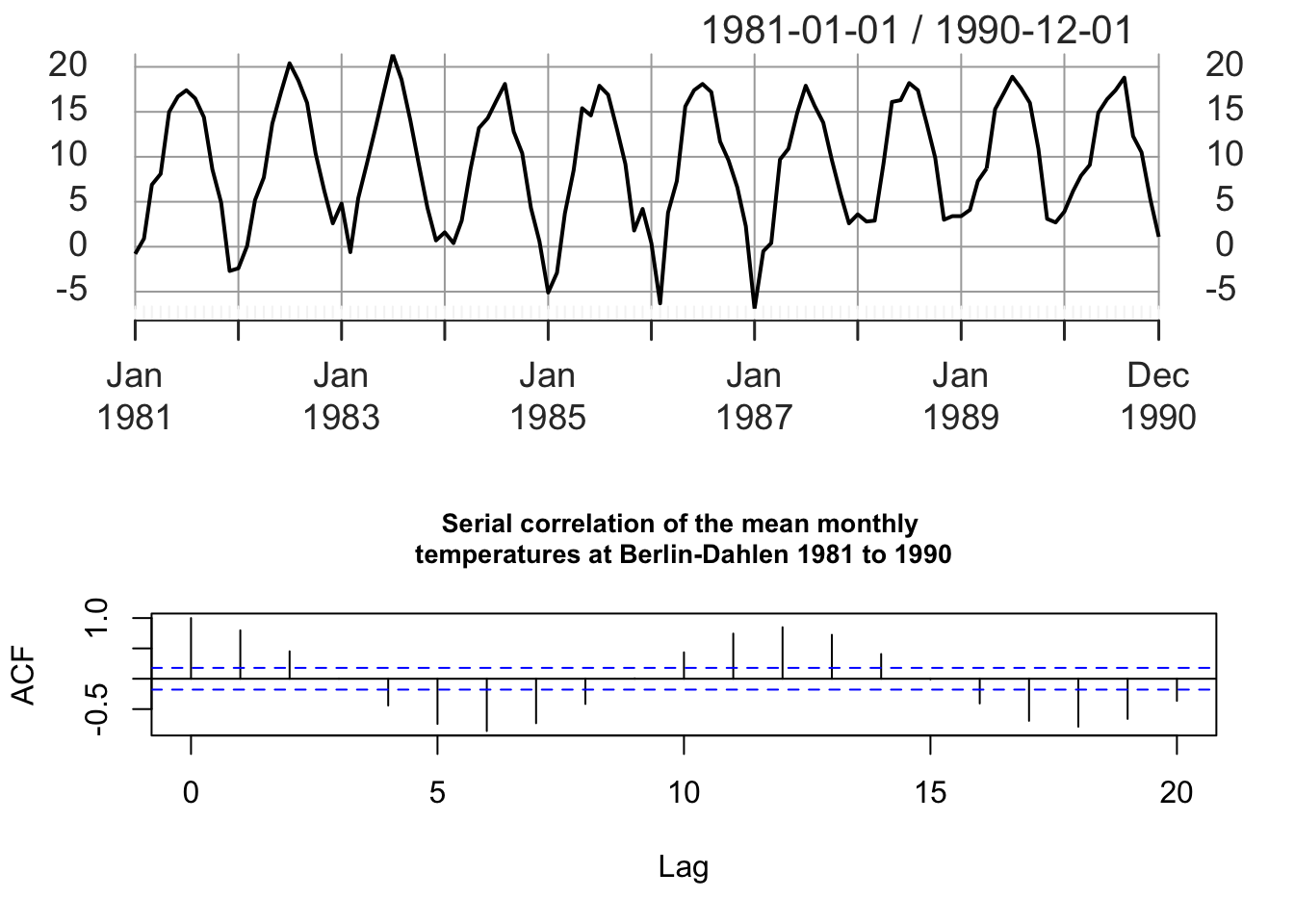We assume that the time series values we observe are the realizations of random variables $$y_1,...,y_t$$, which are in turn part of a larger stochastic process $$\{y_t: t \in \mathbb Z\}$$.

In time series analysis, the analogs to the mean and the variance are the mean function and the autocovariance function.

The mean of a series is defined as

$\mu_t = E(y_t)\text{.}$

The autocovariance function is defined as

$\gamma(s,t) = \text{cov}(y_s, y_t) = E[(y_s-\mu_s)(y_t-\mu_t)]\text{.}$

The autocovariance measures the linear dependence between two points $$(y_s,y_t)$$ at different times. For smooth series the autocovariance function stays large even when the $$s$$ and $$t$$ are far apart, whereas for choppy series the autocovariance function is close to zero for large separations.

If $$s=t$$ it follows that

$\gamma(t,t) = E[(y_t-\mu_t)^2] = \text{var}[y_t] \text{,}$

As in classical statistics, it is more convenient to deal with a measure of association between $$-1$$ and $$1$$. The autocorrelation function (ACF) is computed from the autocovariance function by dividing by the standard deviations of $$y_{s}$$ and $$y_{t}$$.

$\rho(s,t) = \frac{\gamma(s,t)}{\sqrt{(\gamma(s,s)\gamma(t,t))}}$

The autocorrelation, also called serial correlation, is a measure of the internal correlation of a time series. It is a representation of the degree of similarity between the time series and a lagged version of itself. High autocorrelation values mean that the future is strongly correlated to the past.

The cross-covariance function is a measure of predictability of one series $$y_t$$ from another series $$x_s$$.

$\gamma_{xy}(s,t) = \text{cov}(x_s, y_t) = E[(x_{s}-\mu_{xs})(y_{t}-\mu_{yt})]$

The cross-covariance function can be scaled to $$[1,-1]$$, referred to as cross-correlation function (CCF).

$\rho_{xy}(s,t) = \frac{\gamma_{xy}(s,t)}{\sqrt{(\gamma_x(s,s)\gamma_y(t,t))}}$

#### Estimation of Correlation

The real values for the mean and the autocorrelation function are in general not known and must be estimated based on the sample data $$y_1, y_2, ...y_n$$.

The mean function is estimated by the sample mean

$\bar y = \frac{1}{n}\sum_{t=1}^ny_t\text{,}$

and the theoretical autocorrelation function is estimated by the sample ACF

$\hat{\rho}(k) = \frac{\hat{\gamma}(k)}{\hat{\gamma}(0)} = \frac{\sum_{t=1}^{n-k}(y_{t+k}-\bar y)(y_t-\bar y)}{\sum_{t=1}^n(y_t-\bar y)^2}\text{,}$ for $$k=0,1,...,n-1$$.

#### The Correlogram

One of the most useful descriptive tools in time series analysis is the correlogram plot which is a plot of the serial correlations $$\hat{\rho}(k)$$ versus the lag $$k$$ for $$k = 0, 1,...,M$$, where $$M$$ is usually much less than the sample size $$n$$.

For the sake of demonstration we consider the monthly temperature times series at the weather station Berlin-Dahlem (ts.FUB.monthly) for the period 1981 to 1990. First, we subset the original time series and then we apply the acf() function to compute the autocorrelation function. Note that by calling the acf() a graph will be plotted by default. Add the additional argument plot = FALSE to the acf() function call to change the default settings.

 # load the data set
library(xts)
## Loading required package: zoo
##
## Attaching package: 'zoo'
## The following objects are masked from 'package:base':
##
##     as.Date, as.Date.numeric
load(url("https://userpage.fu-berlin.de/soga/300/30100_data_sets/DWD_FUB.RData"))

ts.FUB.monthly.1980 <- ts.FUB.monthly["1981/1990"]
par(mfrow = c(2,1))
plot(ts.FUB.monthly.1980,
main = NA,
cex = 0.85,
cex.main = 0.85)
acf(ts.FUB.monthly.1980, main = NA)
title("Serial correlation of the mean monthly \ntemperatures at Berlin-Dahlen 1981 to 1990",
cex.main = 0.85)The correlogram shows an oscillating autocorrelation structure with very strong autocorrelations at a lag of 6 months and multiples of 6. This is to be expected due to the nature of the temperature time series. The blue lines in the correlogram indicate the 95% confidence limits for what can be expected under the hypothesis of white noise.

However, please note that typically trends and periodicities are removed from the data before investigating the autocorrelational structure of the data.

#### Stationarity and non-stationarity time series

A key idea in time series analysis is that of stationarity. Stationarity is considered as an important precondition for the analysis of the correlational structure in the time series. A time series is considered stationary if its behavior does not change over time. This means, for example, that the values always tend to vary about the same level and that their variability is constant over time.

A time series $$\{y_t: t \in \mathbb Z\}$$ is said to be strictly stationary if for any $$k > 0$$ and any $$t_1,...,t_k \in \mathbb Z$$, the distribution of $$(y_{t_1}, ...,y_{t_k})$$ is the same as that for $$(y_{t_1+u}, ...,y_{t_k+u})$$ for every value of $$u$$. Following this definition the stochastic behavior of the process does not change through time.

A weaker definition of stationarity is second order stationarity, also referred to as wide-sense stationarity or covariance stationary. Here, we do not assume anything about the joint distribution of the random responses $$y_{t1}, y_{t2}, y_{t3},...$$ except that the mean is constant $$E[y_t] = \mu$$ and that the covariance between two observations $$y_t$$ and $$y_{t+k}$$ depends only on the lag $$k$$ between two observations and not on the point $$t$$ in the time series.

The theory for time series is based on the assumption of second-order stationarity. However, real-life data are often not stationary. The assumptions of stationarity above only applies after any trends/seasonal effects have been removed.